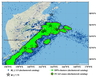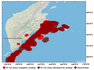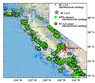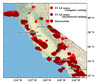RUSSIAN JOURNAL OF EARTH SCIENCES, VOL. 20, ES6010, doi:10.2205/2020ES000754, 2020

# FCAZ-recognition based on declustered earthquake catalogs

B. A. Dzeboev1,2, J. K. Karapetyan3, G. A. Aronov4, B. V. Dzeranov1,2, D. V. Kudin1, R. K.  Karapetyan3, E. V. Vavilin1

1Geophysical Center RAS (GC RAS), Moscow, Russia

3Institute of Geophysics and Engineering Seismology after A. Nazarov, Gyumri, Armenia

4Centre of Geophysical Monitoring NAS of Belarus, Minsk, Belarus

### Abstract

The article presents the results of FCAZ-recognition of the strongest ($M \ge$ 7.75) earthquake-prone areas on the Pacific coast of the Kamchatka Peninsula and strong ($M \ge$ 6.5) earthquake-prone areas in California. For the first time, earthquake epicenters from declustered catalogs were used as recognition objects. Based on the example of the considered regions it is shown that the presence of foreshock and aftershock sequences in the earthquake catalogs does not significantly affect the results of FCAZ-recognition based on the clustering study of weak earthquake epicenters.

### Introduction

In the early 2010s, at the Geophysical Center of the Russian Academy of Sciences, as a result of research by A. D. Gvishiani, S. M. Agayan, and B. A. Dzeboev on the basis of Discrete Mathematical Analysis (DMA) [Gvishiani et al., 2008, 2010; Agayan et al., 2018], the system-analytical [Zgurovsky and Pankratova, 2007] method FCAZ (Formalized Clustering And Zoning) was created [Gvishiani et al., 2013, 2016]. FCAZ makes it possible to effectively recognize areas prone to the strongest, strong, and significant earthquakes based on clustering studies (topological filtration) of the weak earthquake epicenters [Gvishiani et al., 2016, 2020]. Thus, earthquake epicenters starting from a certain magnitude threshold are used as objects for FCAZ-recognition. The results of FCAZ-recognition can be used to solve the problem of seismic hazard assessment [Akopian et al., 2017].

The FCAZ method represents a sequential application of two algorithms: the clustering algorithm/topological filtering algorithm DPS (Discrete Perfect Sets) [Agayan et al., 2014; Gvishiani et al., 2013, 2016] and the E$^2$XT algorithm [Gvishiani et al., 2013, 2016]. DPS selects subsets $W(\alpha (\beta ))$ in a finite set of recognition objects $W$ with a density level $\alpha (\beta )$, where $\beta$ is the optimal value of the maximum density of DPS clusters, which allows one to separate dense clusters of objects $W(\alpha (\beta ))$ from their non-empty complement. The E$^2$XT algorithm implements a formalized single-valued transformation of DPS clusters of earthquake epicenters into nonzero measure flat zones, inside and at the boundaries of which earthquakes can occur. A detailed description of the FCAZ method is given in [Gvishiani et al., 2013, 2016].

Previously, the FCAZ method has proven itself well in recognition of areas prone to strongest, strong, and significant earthquakes in the Andes mountain belt of South America [Gvishiani et al., 2016], on the Pacific coast of the Kamchatka Peninsula [Dzeboev et al., 2018a], in California [Dzeboev et al., 2018b], in the Baikal-Cisbaikalia regions [Gvishiani et al., 2017a] and Altai-Sayan [Gvishiani et al., 2018], in the Caucasus [Gvishiani et al., 2013, 2016], as well as on the Crimean Peninsula and northwest of the Caucasus [Gvishiani et al., 2017b]. The reliability of the FCAZ results is substantiated by the results of control experiments [Gvishiani et al., 2016], the consistency of the FCAZ zones with the epicenters of the earthquakes that have occurred, and by comparing them with the high seismicity zones recognized by the classical EPA (Earthquake-Prone Areas) method [Gelfand et al., 1972, 1976; Gorshkov et al., 2000, 2002, 2003; Soloviev et al., 2016; Gorshkov and Novikova, 2018; Kossobokov and Soloviev, 2018; Gvishiani et al., 2020].

The present paper considers the contribution of foreshock and aftershock sequences [Baranov et al., 2019] to the formation of the final result of determining high seismicity zones by the FCAZ method. For this purpose for the first time epicenters from declustered earthquake catalogs were used as FCAZ-recognition objects. The study was carried out in California and on the Pacific coast of the Kamchatka Peninsula.

### FCAZ-recognition on the Pacific Coast of the Kamchatka Peninsula

In 2018, in [Dzeboev et al., 2018a], the FCAZ method was used to recognize areas prone to the strongest ($\ge$ 7.75) earthquakes on the Pacific coast of the Kamchatka Peninsula. All epicenters of earthquakes with a magnitude $M_L$ $\ge$ 3.5 (44,113 events) and a depth of hypocenters not exceeding 70 km for the period 1962–2015 were used as recognition objects ($M_L$ – Kamchatka regional magnitude [Abubakirov et al., 2018]) [http://www.emsd.ru /sdis/earthquake/catalogue/catalogue.php]. To select the depth of the hypocenters, the distribution histogram of events over depth was constructed [Dzeboev et al., 2018a], which showed that the overwhelming majority of hypocenters fall on the 0–70 km layer, which is consistent with the results of [Levina et al., 2013]. It should be noted that to carry out work on the long-term forecasting of the strongest earthquakes by the method of academician S.A. Fedotov earthquakes with a hypocenter depth of 0–80 km are used [Fedotov and Solomatin, 2015].

It should be noted that the reference regional magnitude scale $M_L$ for earthquakes in Kamchatka and the Commander Islands [Skorkina, 2020], in contrast to the classical magnitude scales, does not have an independent character [Abubakirov et al., 2018]. It is obtained from the values of the Fedotov energy class [Fedotov, 1972] by recalculation according to the Gordeev formula [Gordeev et al., 2006]. In [Abubakirov et al., 2018], the average relationship between the moment magnitude $M_W$ and the Kamchatka regional magnitude $M_L$ was determined for the range $M_W$ = 3.0–6.0 (or $M_L$ = 3.4–6.4): $M_W=M_L-0.4$.Figure 1

In this paper, the Zaliapin-Ben-Zion approach [Zaliapin and Ben-Zion, 2013] was used to decluster the catalog of earthquakes on the Pacific coast of the Kamchatka Peninsula (1962–2015). The resulting declustered catalog contains 24,937 earthquake epicenters with $M_L$ $\ge$ 3.5 and h $\le$ 70 km. Assessment of completeness showed that the magnitude $M_L$ = 3.5 is representative. For this reason, in the present article, the epicenters of earthquakes with $M_L$ $\ge$ 3.5 from the declustered catalog were used as FCAZ-recognition objects. The location of the epicenters of such earthquakes on the region map is shown in Figure 1 with blue and green dots.

The DPS algorithm was applied to the set of earthquake epicenters from the declustered catalog with $M_L$ $\ge$ 3.5. As in the case of the complete catalog recognition [Dzeboev et al., 2018a], two iterations of DPS-clustering were performed. Initially, the algorithm with the density level $\alpha_1(\beta_1)$ was applied. The resulting dense set $W_1(\alpha_1(\beta_1))$ of earthquake epicenters with $M_L$ $\ge$ 3.5 was declared the result at the first iteration of DPS-clustering and was removed from further consideration. After that, the algorithm was applied a second time to the remaining subset $W_2=W \backslash W_1(\alpha_1(\beta_1))$ the density level $\alpha_2(\beta_2)$. This gave new DPS clusters of epicenters $W_2(\alpha_2(\beta_2))$. All objects of recognition included in $W_1(\alpha_1(\beta_1)) \cup W_2(\alpha_2(\beta_2))$ were declared the sought DPS-clusters. Subsequent iterations are performed in a similar way, if necessary.

The calculated values of the maximum density $\beta$ of recognized DPS-clusters and the radius $r$ of localization at iterations are as follows: $\beta_{1}=-0.2$ and $\beta_{2}=-0.2$, $r_{1} = 34.47$ km and $r_{2}=37.6$ km. Notice that the values of the $\beta$ parameter are calculated automatically by the artificial intelligence unit [Gvishiani et al., 2016]. 76% of the considered recognition objects were included in the DPS-clusters. The recognized DPS-clusters are shown in Figure 1 in green.

The E$^2$XT algorithm was applied to DPS-clusters. The optimal values of its input parameters calculated in automatic mode are $\omega = -3.75$ and $v=-2.0$. In this case, the step of the geographic grid is 0.05$\mbox{°}$. In Figure 1, a combination of green and brown colors shows the recognized high seismicity FCAZ-zones.

It should be specially noted here that, despite the almost two-fold decrease in the number of recognition objects, the values of the $\beta, r, \omega, v$ parameters and the percentage of objects included in the DPS-clusters, calculated during recognition based on the declustered catalog, differs very slightly from the values obtained when recognizing from the complete catalog of earthquakes [Dzeboev et al., 2018a]. We remind that the following values were obtained during recognition based on the complete catalog [Dzeboev et al., 2018a]: $\beta_{1}=-0.15, \beta_{2}=-0.2, r_{1} = 30.9$ km, $r_{2}=33.7$ km, $\omega=-4, v=-2.25$ and 73.3% of objects were included to DPS-clusters. This may indicate rather close spatial distributions of earthquake epicenters in the considered catalogs (complete catalog and declustered catalog).

In Figure 1, black asterisks show the epicenters of earthquakes with $M \ge 7.75$ known (since 1900) in the considered region. The catalog of such strongest earthquakes that are used in the present work is given in [Dzeboev et al., 2018a]. As seen from Figure 1, 7 (87.5%) of the 8 considered earthquakes with $M \ge 7.75$ fall inside the FCAZ-zones. It should be noted that FCAZ-zones (Figure 1) contain 77.2% of earthquakes with $M_L$ $\ge$ 4.5 from those available in the instrumental declustered catalog used for recognition. The FCAZ-zones occupy about 44.5% of the seismically active Kuril-Kamchatka and Aleutian arcs (Figure 1). All this makes it possible with a high degree of reliability to interpret the recognized FCAZ-zones (Figure 1) as earthquake-prone areas with M $\ge 7.75$ on the Pacific coast of the Kamchatka Peninsula.

Explaining the reasons for not falling into the recognized FCAZ-zones of the epicenter of the Ozernovsky earthquake that occurred on October 20, 1963, with $M=7.8$ (6 in Figure 1) it is necessary to say the following. This earthquake occurred outside the zone of modern subduction and the conditions for its occurrence are fundamentally different from other considered earthquakes. This is also substantiated by the fact that the epicenter of the Ozernovsky earthquake is located outside the territory for which the long-term forecast of the strongest earthquakes by the method of Academician S.A. Fedotov is performed [Fedotov and Solomatin, 2015].

To check the reliability of the results of FCAZ-recognition based on the declustered catalog of earthquakes, control computational experiments "individual seismic history" and "complete seismic history" were carried out [Gvishiani et al., 2016]. The experimental results should be considered successful. This speaks in favor of the reliability of the FCAZ-zones interpretation (Figure 1) as areas prone to the strongest earthquakes on the Pacific coast of the Kamchatka Peninsula.Figure 2

Figure 2 shows a comparison of FCAZ-zones of earthquake-prone areas with $M \ge 7.75$ on the Pacific coast of the Kamchatka Peninsula, identified using epicenters from the complete [Dzeboev et al., 2018a] and declustered (Figure 1) earthquake catalogs. Analysis of Figure 2 shows that FCAZ-zones recognized from different catalogs coincide quite well. The area of zones recognized using epicenters from the declustered catalog is 12% larger than the area of zones recognized based on the complete catalog. The ratio of the intersection area of zones to the area of their union is 0.77. It should be noted that the zones recognized from the declustered catalog represent a single structure along the entire Pacific coast of Kamchatka. Unlike the FCAZ-zones recognized from the complete catalog, there are no zones on the coast of the peninsula, in particular, in the Avacha Bay area. The high seismicity zone located east of Kronotsky Bay (Figure 1 in [Dzeboev et al., 2018a]) moved further into the ocean (Figure 1, Figure 2). It has to be mentioned that the FCAZ-zones recognized in [Dzeboev et al., 2018a] and in the present article have almost identical areas and contain 7 of the 8 considered strongest earthquakes in the region.

### FCAZ-recognition in California

Recognition of strong ($M \ge 6.5$) earthquake-prone areas in California by the FCAZ method was carried out in 2018 in [Dzeboev et al., 2018b]. The epicenters of 31,874 crustal [Bondur et al., 2017] earthquakes with $M \ge$ 3.0 [http://www.ncedc.org/ anss/catalog-search.html] for the period 1960–2012 were used as recognition objects.Figure 3

In the present paper, the Zaliapin-Ben-Zion approach [Zaliapin and Ben-Zion, 2013] was used to decluster the California earthquake catalog. The resulting declustered catalog contains 10,176 earthquakes with $M \ge 3.0$. Assessment of the completeness magnitude $M_c$ of catalog showed that $M=3.0$ is representative. For this reason, the epicenters of earthquakes with $M \ge 3.0$ were used as objects for FCAZ-recognition. The location of such recognition objects on the California map is shown in Figure 3 blue and green dots.

The DPS algorithm was applied to a set of recognition objects. As in the case of recognition based on the complete catalog [Dzeboev et al., 2018b], three DPS-clustering iterations were performed. The calculated optimal values of the maximum density $\beta$ of the recognized DPS-clusters and the radius $r$ of localization at iterations: $\beta_1=0.2$, $\beta_2$ =$-0.05$, $\beta_3=0.05$, $r_1=21.84$ km, $r_2=40.02$ km, $r_3=39.39$ km. The DPS clusters of weak earthquake epicenters formed in this way include 61% of recognition objects and are shown in Figure 3 in green.

The E$^2$XT algorithm was applied to DPS-clusters. The optimal values of its input parameters calculated in the automatic mode are: $\omega = -5.0$ and $v=-0.2$. In this case, the step of the geographic grid is 0.05$\mbox{°}$. FCAZ-zones are shown in Figure 3 with a combination of green and brown colors. It should be mentioned that the recognized FCAZ-zones contain 64.5% of the epicenters of earthquakes with $M \ge$ 4.5 from those available in the used instrumental declustered catalog.

It should be noted here that, in contrast to the recognition described in the previous paragraph, in Kamchatka and California, the calculated values of the FCAZ parameters for the case of the declustered catalog differ markedly from the values of the same parameters calculated for the case of the complete catalog [Dzeboev et al., 2018b]. This can be explained by the fact that after declustering the catalog, the number of recognition objects decreased by 68%, which led to a change in the quantitative-spatial distribution of the set of recognition objects. At the same time, the results presented below show that this did not lead to a significant change in both the formed DPS-cluster and in fact recognized FCAZ-zones.

In Figure 3 black asterisks show the epicenters of all known earthquakes in California with $M \ge$ 6.5 that occurred up to 2012 inclusively (i.e., before the end date of the instrumental earthquake catalog used for recognition). The catalog of these strong earthquakes is given in [Dzeboev et al., 2018b]. Figure 3 shows that the recognized FCAZ-zones are in fairly good agreement with the epicenters of 33 earthquakes with $M \ge$ 6.5 that occurred before 2012. Outside the FCAZ-zones, there are epicenters 4, 5, 17, 18, and 31 that were not included in the recognized zones and complete catalog recognition [Dzeboev et al., 2018b]. The only deterioration in the consistency of FCAZ-zones and earthquakes with $M \ge$ 6.5 is epicenter 29, which is located near the boundary of FCAZ-zones (Figure 3), recognized based on the declustered catalog.

To check the reliability of the results of FCAZ-recognition based on the declustered catalog of earthquakes, control computational experiments "individual seismic history" and "complete seismic history" were carried out [Gvishiani et al., 2016]. The experimental results were successful. This speaks in favor of the reliability of the FCAZ-zones interpretation shown in Figure 3, as earthquake-prone areas with $M \ge$ 6.5 in California.

The best validation for the reliability of the results for FCAZ-recognition of high seismicity zones is to conduct a pure experiment, i.e. analysis of the location of the epicenters of earthquakes that occurred after the end of the instrumental catalog used for recognition, relative to these zones. After 2012, two earthquakes with $M \ge$ 6.5 occurred in California. The first earthquake occurred on March 10, 2014, with $M$ = 6.8. Its epicenter is shown in Figure 3 yellow asterisk and is located in the northwest of the region in the Pacific Ocean within the FCAZ zone. The second earthquake occurred on July 6, 2019, and had a magnitude $M$ = 7.1. The epicenter of this earthquake is also located inside the FCAZ-zones and is shown by a red asterisk in Figure 3 . Thus, the result of the pure experiment should be recognized as successful.Figure 4

Figure 4 shows a comparison of FCAZ-zones of earthquake-prone areas with $M \ge$ 6.5 in California, recognized using epicenters from the complete [Dzeboev et al., 2018b] and declustered (Figure 3) earthquake catalogs.

Analysis of Figure 4 shows that FCAZ-zones recognized in California using the complete and declustered earthquake catalogs have the same area. The ratio of the total area of zones recognized using epicenters from the declustered catalog to the total area of zones recognized based on the complete catalog is 0.9937. In this case, the ratio of the intersection area of FCAZ-zones recognized from different catalogs to the area of their union is 0.69. The main differences between FCAZ-zones are observed in the south, east, and north of the Sierra Nevada mountains (some of these differences are located in the state of Nevada), as well as in the north of the considered California region in the ocean. Note that the FCAZ-zones from [Dzeboev et al., 2018b] and shown in Figure 3, have equal areas and contain, respectively, 30 and 29 out of 35 considered strong earthquakes in the region.

### Conclusions

Earthquake-prone areas on the Pacific coast of the Kamchatka Peninsula and in California recognized using as objects of all earthquake epicenters [Dzeboev et al., 2018a, 2018b] and earthquake epicenters from the same, but declustered catalogs (Figure 1 and Figure 3) turned out to be almost the same (Figure 2 and Figure 4). The optimal values of the $\beta$ parameter calculated in the automatic mode (the maximum density in recognized DPS-clusters, and in fact, the algorithm's "Viewpoint" at the topology of a set of objects and the separability of their dense clusters from a loose complement) for both recognitions in Kamchatka turned out to be very close: $- 0.2$ and $-0.2$ for a declustered catalog (Figure 1); $-0.15$ and $-0.2$ for the complete catalog [Dzeboev et al., 2018a].

The results of the paper suggest that the presence of foreshock and aftershock sequences in the earthquake catalogs does not significantly affect the final results of FCAZ-recognition based on clustering (topological filtration) study of the weak earthquake epicenters.

#### Acknowledgments

The reported study was funded by RFBR, project number 20-35-70054 "Systems approach to recognition algorithms for seismic hazard assessment". This work employed data provided by the Shared Research Facility "Analytical Geomagnetic Data Center" of the Geophysical Center of RAS .

### References

Abubakirov, I. R., A. A. Gusev, E. M. Guseva, at al. (2018) , Mass determination of moment magnitudes $M_w$ and establishing the relationship between $M_w$ and $M_L$ for moderate and small Kamchatka earthquakes, Izv., Phys. Solid Earth, 54, p. 33–47, https://doi.org/10.1134/S1069351318010019.

Agayan, S., Sh. Bogoutdinov, M. Dobrovolsky (2014) , Discrete perfect sets and their application in cluster analysis, Cybernetics and Systems Analysis, 50, no. 2, p. 176–190, https://doi.org/10.1007/s10559-014-9605-9.

Agayan, S., Sh. Bogoutdinov, R. Krasnoperov (2018) , Short introduction into DMA, Russ. J. Earth. Sci., 18, p. ES2001, https://doi.org/10.2205/2018ES000618.

Akopian, S., V. Bondur, E. Rogozhin (2017) , Technology for monitoring and forecasting strong earthquakes in Russia with the use of the seismic entropy method, Izv., Phys. Solid Earth, 53, p. 32–51, https://doi.org/10.1134/S1069351317010025.

Baranov, S. V., A. D. Gvishiani, C. Narteau, P. N. Shebalin (2019) , Epidemic type aftershock sequence exponential productivity, Russ. J. Earth. Sci., 19, p. ES6003, https://doi.org/10.2205/2019ES000695.

Bondur, V. G., I. A. Garagash, M. B. Gokhberg (2017) , The dynamics of the stress state in Southern California based on the geomechanical model and current seismicity: Short term earthquake prediction, Russ. J. Earth. Sci., 17, p. ES1005, https://doi.org/10.2205/2017ES000596.

Dzeboev, B. A., S. M. Agayan, Yu. I. Zharkikh, et al. (2018a) , Strongest Earthquake-Prone Areas in Kamchatka, Izv., Phys. Solid Earth, 54, no. 2, p. 284–291, https://doi.org/10.1134/S1069351318020052.

Dzeboev, B. A., R. I. Krasnoperov, I. O. Belov, et al. (2018b) , Modified algorithmic system FCAZm and strong earthquake-prone areas in California, Geoinformatika, no. 2, p. 2–8 (in Russian).

Fedotov, S. A. (1972) , Energy Classification of the Kuril–Kamchatka Earthquakes and the Problem of Magnitudes, 116 pp., Nauka, Moscow (in Russian).

Fedotov, S. A., A. V. Solomatin (2015) , The long-term earthquake forecast for the Kuril-Kamchatka island arc for the September 2013 to August 2018 period; the seismicity of the arc during preceding deep-focus earthquakes in the sea of Okhotsk (in 2008, 2012, and 2013 at $M$ = 7.7, 7.7, and 8.3), J. Volcanolog. Seismol., 9, p. 65–80, https://doi.org/10.1134/S0742046315020025.

Gelfand, I. M., Sh. I. Guberman, M.L. Izvekova, et al. (1972) , Criteria of high seismicity determined by pattern recognition, Tectonophysics, 13, no. 1–4, p. 415–422, https://doi.org/10.1016/0040-1951(72)90031-5.

Gelfand, I. M., Sh. I. Guberman, V. I. Keilis-Borok, et al. (1976) , Pattern recognition applied to earthquake epicenters in California, Physics of the Earth and Planetary Interiors, 11, no. 3, p. 227–283.

Gordeev, E. I., A. A. Gusev, V. I. Levina, et al. (2006) , Shallow Earthquakes in Kamchatka, Vulkanol. Seismol., no. 3, p. 28–38 (in Russian).

Gorshkov, A. I., I. V. Kuznetsov, A. A. Soloviev, et al. (2000) , Identification of future earthquake sources in the Carpatho-Balkan orogenic belt using morphostuctural criteria, Pure and Applied Geophysics, 157, no. 1–2, p. 79–95, https://doi.org/10.1007/PL00001101.

Gorshkov, A. I., G. F. Panza, A. A. Soloviev, et al. (2002) , Morphostructural zonation and preliminary recognition of seismogenic nodes around the Adria margin in peninsular Italy and Sicily, Journal of Seismology and Earthquake Engineering, 4, no. 1, p. 1–24.

Gorshkov, A., V. Kossobokov, A. Soloviev (2003) , Recognition of Earthquake-Prone Areas, Nonlinear Dynamics of the Lithosphere and Earthquake Prediction, edited by Keilis-Borok V., Soloviev A., p. 239–310, Springer, Berlin, Heidelberg, https://doi.org/10.1007/978-3-662-05298-3_6.

Gorshkov, A., O. Novikova (2018) , Estimating the validity of the recognition results of earthquake-prone areas using the ArcMap, Acta Geophysica, 66, no. 5, p. 843–853, https://doi.org/10.1007/s11600-018-0177-3.

Gvishiani, A. D., S. M. Agayan, Sh. R. Bogoutdinov (2008) , Discrete mathematical analysis and monitoring of volcanoes, Inzh. Ekol., no. 5, p. 26–31 (in Russian).

Gvishiani, A. D., S. M. Agayan, Sh. R. Bogoutdinov, et al. (2010) , Discrete mathematical analysis and applications in geology and geophysics, Vestnik KRAUNTs. Nauki o Zemle, no. 2, p. 109–125 (in Russian).

Gvishiani, A., B. Dzeboev, S. Agayan (2013) , A new approach to recognition of the earthquake-prone areas in the Caucasus, Izv., Phys. Solid Earth, 49, no. 6, p. 747–766, https://doi.org/10.1134/S1069351313060049.

Gvishiani, A. D., B. A. Dzeboev, S. M. Agayan (2016) , FCAZm intelligent recognition system for locating areas prone to strong earthquakes in the Andean and Caucasian mountain belts, Izv., Phys. Solid Earth, 54, no. 4, p. 461–491, https://doi.org/10.1134/S1069351316040017.

Gvishiani, A. D., B. A. Dzeboev, I. O. Belov (2017a) , Successive Recognition of Significant and Strong Earthquake-Prone Areas: The Baikal-Transbaikal Region, Doklady Earth Sciences, 477. Part 2, p. 1488–1493, https://doi.org/10.1134/S1028334X1712025X.

Gvishiani, A. D., B. A. Dzeboev, N. A. Sergeyeva, et al. (2017b) , Formalized Clustering and the Significant Earthquake-Prone Areas in the Crimean Peninsula and Northwest Caucasus, Izv., Phys. Solid Earth, 53, no. 3, p. 353–365, https://doi.org/10.1134/S106935131703003X.

Gvishiani, A. D., B. A. Dzeboev, N. A. Sergeyeva, et al. (2018) , Significant Earthquake-Prone Areas in the Altai-Sayan Region, Izv., Phys. Solid Earth, 54, no. 3, p. 406–414, https://doi.org/10.1134/S1069351318030035.

Gvishiani, A. D., A. A. Soloviev, B. A. Dzeboev (2020) , Problem of Recognition of Strong-Earthquake-Prone Areas: a State-of-the-Art Review, Izv., Phys. Solid Earth, 56, no. 1, p. 1–23, https://doi.org/10.1134/S1069351320010048.

Kossobokov, V. G., A. A. Soloviev (2018) , Pattern recognition in problems of seismic hazard assessment, Chebyshevskii Sbornik, 19, no. 4, p. 55–90, https://doi.org/10.22405/2226-8383-2018-19-4-55-90 (In Russian).

Levina, V. I., A. V. Lander, et al. (2013) , The seismicity of the Kamchatka region: 1962–2011, J. Volcanolog. Seismol., 7, p. 37–57, https://doi.org/10.1134/S0742046313010053.

Skorkina, A. A. (2020) , Scaling of two corner frequencies of source spectra for earthquakes of the Bering fault, Russ. J. Earth. Sci., 20, p. ES2001, https://doi.org/10.2205/2020ES000704.

Soloviev, A. A., A. I. Gorshkov, A. A. Soloviev (2016) , Application of the data on the lithospheric magnetic anomalies in the problem of recognizing the earthquake prone areas, Izv., Phys. Solid Earth, 52, p. 803–809, https://doi.org/10.1134/S1069351316050141.

Zaliapin, I., Y. Ben-Zion (2013) , Earthquake clusters in southern California I: identification and stability, J. Geophys. Res. Solid Earth, 118, p. 2847–2864, https://doi.org/10.1002/jgrb.50179.

Zgurovsky, M. Z, N. D. Pankratova (2007) , System Analysis: Theory and Applications, 447 pp., Springer-Verlag, Berlin, Heidelberg, https://doi.org/10.1007/978-3-540-48880-4.

Received 29 October 2020; accepted 24 November 2020; published 29 November 2020.Citation: Dzeboev B. A., J. K. Karapetyan, G. A. Aronov, B. V. Dzeranov, D. V. Kudin, R.~K.~ Karapetyan, E. V. Vavilin (2020), FCAZ-recognition based on declustered earthquake catalogs, Russ. J. Earth Sci., 20, ES6010, doi:10.2205/2020ES000754.

Generated from LaTeX source by ELXfinal, v.2.0 software package.